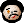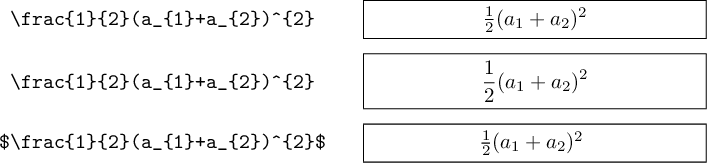# 2015-05-23 Math examples in TeX, part II

Some time ago, I wrote about a macro to typeset examples of math typesetting in LaTeX. I mentioned then that there’s a bit more to the real code I’m using. So, here it is, in hope that someone might find this inspiring and/or learn something from the methods I used.

First of all, I wanted to be able to show examples of displayed equations, in the same format (code to the left, result to the right). All this is about how not to duplicate code, so I obviously did not want to copy-paste (ekhm, kill-yank) the \@mathdemo macro. Here’s the goal: make it general enough so that it’s possible to write \dmathdemo with minimal code duplication.

This is simple. All we need to do is put \displaystyle in front of the equation. And while we’re at it, we might want to demonstrate various math delimiters (like $...$ and $$...$$), so I also wanted \rmathdemo (r’ like raw’), in which I would supply the math delimiters, too. Here’s the code (don’t forget to put \makeatletter/\makeatother around it!):

\def\mathdemo{%
\bgroup
\let\do\@makeother
\dospecials
\global\let\@atbeginmathdemo=$$\global\let\@atendmathdemo=$$
\@mathdemo
}
\def\rmathdemo{% raw
\bgroup
\let\do\@makeother
\dospecials
\global\let\@atbeginmathdemo=\relax
\global\let\@atendmathdemo=\relax
\@mathdemo
}
\def\dmathdemo{%
\bgroup
\let\do\@makeother
\dospecials
\global\def\@atbeginmathdemo{$$\displaystyle} \global\let\@atendmathdemo=$$
\@mathdemo
}
\def\@mathdemo#1{%
\egroup
\par\smallskip\noindent
\makebox[0.48\textwidth]{\texttt{#1}}\hfill
\framebox[0.48\textwidth]{%
\@atbeginmathdemo\scantokens{#1}\@atendmathdemo
}\par\smallskip
}


Here’s how you use these macros.

\mathdemo\frac{1}{2}(a_1+a_2)^2
\dmathdemo\frac{1}{2}(a_1+a_2)^2
\rmathdemo$\frac{1}{2}(a_1+a_2)^2$


and here’s the result:Next time, I will show a few finishing touches – but for today that’s it. Happy TeXing!